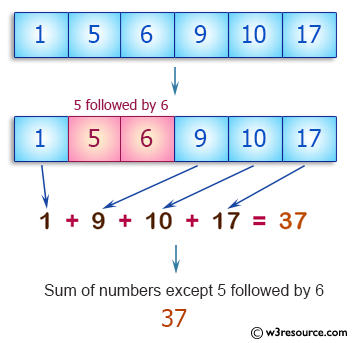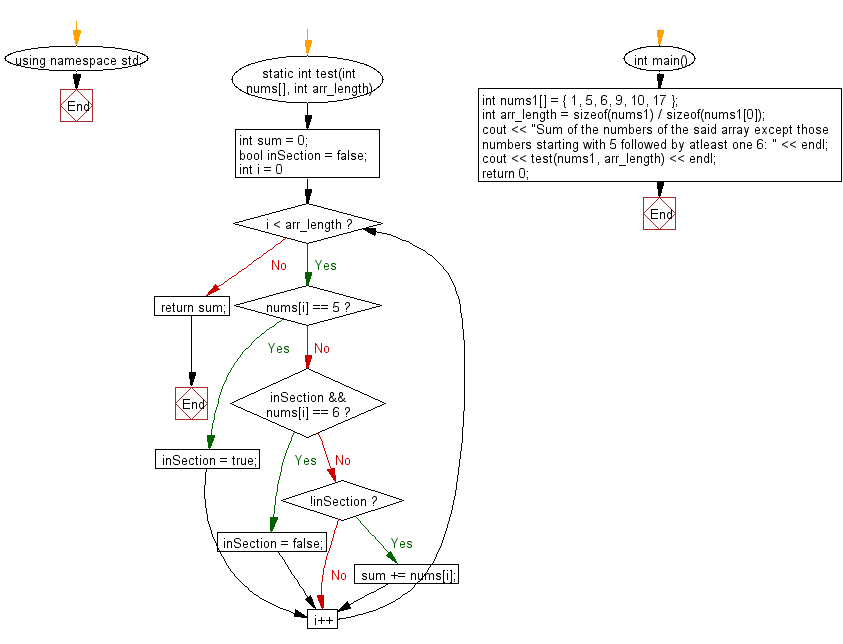﻿ C++ : Sum of the numbers in a array except some numbers# C++ Exercises: Compute the sum of the numbers in a given array except those numbers starting with 5 followed by atleast one 6

## C++ Basic Algorithm: Exercise-101 with Solution

Write a C++ program to compute the sum of the numbers in a given array except those numbers starting with 5 followed by atleast one 6. Return 0 if the given array has no integer.

Sample Solution:

C++ Code :

``````#include <iostream>
using namespace std;
static int test(int nums[], int arr_length)
{
int sum = 0;
int inSection = 0;
int flag = 0;
for (int i = 0; i < arr_length; i++)
{
inSection = 0;
if (nums[i] == 5)
{
inSection = 0;
flag = 1;
}
if (inSection == 0 && nums[i] == 6)
{
if (flag == 1)
{
sum = sum - 5;
inSection = 1;
}
flag = 0;
}
if (inSection == 0)
{
sum += nums[i];
}
}
return sum;
}
int main()
{
int nums1[] = { 1, 5, 9, 10, 17 };
int arr_length = sizeof(nums1) / sizeof(nums1);
cout << "Sum of the numbers of the said array except those numbers starting with 5 followed by atleast one 6: " << endl;
cout << test(nums1, arr_length) << endl;
int nums2[] = { 1, 5, 6, 9, 10, 17 };
arr_length = sizeof(nums2) / sizeof(nums2);
cout << "Sum of the numbers of the said array except those numbers starting with 5 followed by atleast one 6: " << endl;
cout << test(nums2, arr_length) << endl;
return 0;
}
``````

Sample Output:

```Sum of the numbers of the said array except those numbers starting with 5 followed by atleast one 6:
42
Sum of the numbers of the said array except those numbers starting with 5 followed by atleast one 6:
37
```

Pictorial Presentation:Flowchart:C++ Code Editor:

Contribute your code and comments through Disqus.

What is the difficulty level of this exercise?

﻿